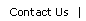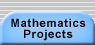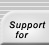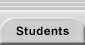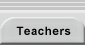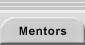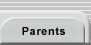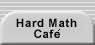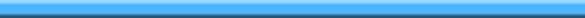# Pascal's Triangle

Pascal's triangle is a triangular array of counting numbers. It begins with a first line of 1. The elements of subsequent rows are found by adding the two elements diagonally above in the preceding row (e.g., 10 is the sum of the 4 and 6 above it). The outermost elements of each row are always 1, because there is no second number above to contribute to the sum.

 1 1 1 1 2 1 1 3 3 1 1 4 6 4 1 1 5 10 10 5 1

There are many ways to derive the values in the triangle. See Ask Dr. Math: FAQ: Pascal's Triangle for a discussion of the triangle and several methods for generating it. See the links from that page to learn more about Pascal's Triangle. Also look at Ladja's Report on Pascal's Triangle and Algebra Through Problem Solving, Chapter 1: The Pascal Triangle.

For help generating pictures of the remainder patterns see:Translations of mathematical formulas for web display were created by tex4ht. © Copyright 2003 Education Development Center, Inc. (EDC)